The GLIMMIX Procedure

### Quasi-likelihood for Independent Data

Quasi-likelihood estimation uses only the first and second moment of the response. In the case of independent data, this requires only a specification of the mean and variance of your data. The GLIMMIX procedure estimates parameters by quasi-likelihood, if the following conditions are met:

• The response distribution is unknown, because of a user-specified variance function.

• There are no G-side random effects.

• There are no R-side covariance structures or at most an overdispersion parameter.

Under some mild regularity conditions, the function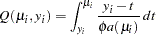known as the log quasi-likelihood of theth observation, has some properties of a log-likelihood function (McCullagh and Nelder 1989, p. 325). For example, the expected value of its derivative is zero, and the variance of its derivative equals the negative of the expected value of the second derivative. Consequently,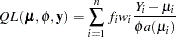can serve as the score function for estimation. Quasi-likelihood estimation takes as the gradient and "Hessian" matrix—with respect to the fixed-effects parameters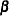—the quantities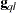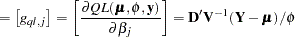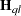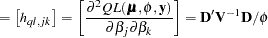In this expression,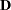is a matrix of derivatives of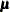with respect to the elements in, and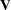is a diagonal matrix containing variance functions,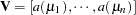. Notice that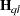is not the second derivative matrix of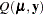. Rather, it is the negative of the expected value of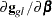.thus has the form of a "scoring Hessian."

The GLIMMIX procedure fixes the scale parameter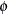at 1.0 by default. To estimate the parameter, add the statement

```    random _residual_;
```

The resulting estimator (McCullagh and Nelder 1989, p. 328) is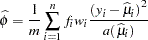where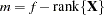if the NOREML option is in effect,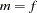otherwise, and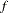is the sum of the frequencies.

See Example 38.4 for an application of quasi-likelihood estimation with PROC GLIMMIX.Previous Page | Next Page | Top of Page